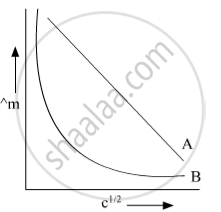# In the Plot of Molar Conductivity (∧M) Vs Square Root of Concentration (C1/2) Following Curves Are Obtained for Two Electrolytes a and B : - Chemistry

#### Question

Short Note

In the plot of molar conductivity (∧m) vs square root of concentration (c1/2) following curves are obtained for two electrolytes A and B :(i) predict the nature of electrolytes A and B.
(ii) What happens on the extrapolation of ∧m to concentration approaching for electrolytes A and B?

#### Solution

Electrolyte A is strong electrolyte & Electrolyte B is weak electrolyte On extrapolation of Λm to concentration approaching zero for strong electrolytes, we get the value of Λ= i.e. molar conductance at infinite dilution In the case of weak electrolytes, Λm increases steeply on dilution. Therefore,  Λ=m cannot be obtained by extrapolation.

Is there an error in this question or solution?